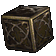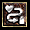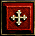(差异) ←上一修订 | 最新修订 (差异) | 下一修订→ (差异)

## 赫拉迪克方块赫拉迪克方块也叫做赫拉迪克宝盒.可以通过完成第II场景的赫拉迪克之杖任务获得,掉落于利爪蝮蛇神殿的第3层:亡者大厅,它还可以帮助你完成后面的一些任务. 同时它还提供了额外的储物空间和合成物品等新的功能.赫拉迪克方块在你的物品栏中提供了12格的空间. 但是它本身只占用 2x2 的空间.当你拣起物品后,可以将它们直接放入盒子 ,当物品放入时,会有声音提示.如果你要取出其中的物品,可以鼠标右键点击,然后取出物品.一些物品在盒子中是可以直接使用的,例如 城镇传送之书.当凯恩辨视未鉴定的物品时,会连同盒子内的物品一同鉴定,所以当你有想保持未鉴定状态的物品时,请不要放在盒子内.你不能将一个盒子放进另一个盒子中, 如此循环.....可以将一些带有抗性的戒指项链等小物品放在盒子内,当你面对某种元素攻击的头目时,可以随时拿出使用.

###合成公式3 个相同符文 (符文 1-9#) = 1 个更高级别符文

3 艾尔 -> 1 艾德

3 艾德 -> 1 特尔

3 特尔 -> 1 那夫

3 那夫 -> 1 爱斯

3 爱斯 -> 1 伊司

3 伊司 -> 1 塔尔

3 塔尔 -> 1 拉尔

3 拉尔 -> 1 欧特

3 欧特 -> 1 书尔3 完美的骷髅 + 1 稀有装备 + 乔丹之石 = 给该稀有装备增加 1 凹槽1 完美的骷髅 + 1 稀有装备 + 乔丹之石 = 1 同类型更高品质的稀有装备6 完美的骷髅 + 1 稀有装备 = 1 随机新的同类型稀有装备4 治疗药剂 (任何类型) + 红宝石 (任何类型) + 魔法剑 = 吸血属性的魔法剑3 魔法戒指 = 1 随机魔法项链3 魔法项链 = 1 随机魔法戒指3 回复活力药剂 = 1 全面回复活力药剂3 同类型宝石 (完美的 级别之下) = 1 高一级同类型宝石1 长矛 + 1 箭矢 = 1 标枪1 斧头 + 1 匕首 = 1 飞斧3 完美的宝石 (任何类型) + 1 魔法物品 = 1 相同类型新的魔法物品窒息瓦斯药剂 + 任何类型治疗药剂 = 1 解毒药剂6 完美的宝石 (1 每种类型) + 1 项链 (魔法) = 所有抗性项链1 魔法戒指 + 1 完美的绿宝石 + 1 解毒药剂 = 1 抗毒戒指1 魔法戒指 + 1 完美的红宝石 + 1 爆裂药剂 = 1 抗火戒指1 魔法戒指 + 1 完美的黄宝石 + 1 回复活力药剂 = 1 抗电戒指1 魔法戒指 + 1 完美的蓝宝石 + 1 溶解药剂 = 1 抗冰戒指1 魔法盾牌 (任何类型) + 1 狼牙棒 (任何质量) + 2 骷髅 (任何质量) = 尖刺之魔法盾牌 ilvl=30.钻石 (任何品质) + 1 法杖 (任何类型及品质) + 1 波形刀 (任何品质) + 1 腰带 (任何类型及品质) = 野人的前缀的木棍类型武器ivl = 50.

###1.10 版合成公式3 书尔 + 1 碎裂的黄宝石 = 安姆3 安姆 + 1 碎裂的紫宝石 = 索尔3 索尔 + 1 碎裂的蓝宝石 = 夏3 夏 + 1 碎裂的红宝石 = 多尔

#### 以下合成公式只能作用在单机角色,开放角色 或是 天梯角色. 不能作用于服务器上的普通角色人物.3 多尔 + 1 碎裂的绿宝石 = 海尔3 海尔 + 1 碎裂的钻石 = 破3 破 + 1 裂开的黄宝石 = 卢姆3 卢姆 + 1 裂开的紫宝石 = 科3 科 + 1 裂开的蓝宝石 = 法尔3 法尔 + 1 裂开的红宝石 = 蓝姆3 蓝姆 + 1 裂开的绿宝石 = 普尔2 普尔 + 1 裂开的钻石 = 乌姆2 乌姆 + 1 黄宝石 = 马尔2 马尔 + 1 紫宝石 = 伊司特2 伊司特 + 1 蓝宝石 = 古尔2 古尔 + 1 红宝石 = 伐克斯2 伐克斯 + 1 绿宝石 = 欧姆2 罗 + 1 无瑕疵的黄宝石 = 瑟2 瑟 + 1 无瑕疵的紫宝石 = 贝2 贝 + 1 无瑕疵的蓝宝石 = 乔2 乔 + 1 无瑕疵的红宝石 = 查姆2 查姆 + 1 无瑕疵的绿宝石 = 萨德3 治疗药剂 + 3 法力药剂 + 1 碎裂的宝石 = 1 回复活力药剂3 治疗药剂 + 3 法力药剂 + 1 宝石 (第三级) = 1 全面回复活力药剂3 标准宝石 (普通) + 1 有凹槽的武器 (任何类型) = 1 有凹槽的魔法武器 (相同类型)3 碎裂的宝石 + 1 魔法武器 = 有凹槽的魔法武器 在魔法武器上加入凹槽.3 无瑕疵的宝石 + 1 魔法武器 = 有凹槽的魔法武器 在魔法武器上加入凹槽.1 塔尔 + 1 书尔 + 1 完美的黄宝石 + 普通级盔甲 = 同样类型有凹槽的盔甲 凹槽数量会发生变化.1 拉尔 + 1 安姆 + 1 完美的紫宝石 + 普通级武器 = 同样类型有凹槽的武器 凹槽数量会发生变化.1 拉尔 + 1 書尔 + 1 完美的蓝宝石 + 普通级头盔 = 同样类型有凹槽的头盔 凹槽数量会发生变化.1 塔尔 + 1 安姆 + 1 完美的红宝石 + 普通级盾牌 = 同样类型有凹槽的盾牌 凹槽数量会发生变化.1 艾德 + 1 碎裂的宝石 + 劣质的武器 = 同样类型普通品质的武器 ilvl = 1. ilvl 始终被设定为 1.1 艾尔 + 1 碎裂的宝石 + 劣质的盔甲 = 同样类型普通品质的盔甲1 拉尔 + 1 索尔 + 1 完美的绿宝石 + 普通级暗金武器 = 扩展级暗金武器1 塔尔 + 1 夏 + 1 完美的钻石 + 普通级暗金盔甲 = 扩展级暗金盔甲1 卢姆 + 1 普尔 + 1 完美的绿宝石 + 扩展级暗金武器 = 精华级暗金武器 (单机游戏, 天梯模式独有)1 科 + 1 蓝姆 + 1 完美的钻石 + 扩展级暗金盔甲 = 精华级暗金盔甲 (单机游戏, 天梯模式独有)1 欧特 + 1 安姆 + 1 完美蓝宝石 + 普通级杰出武器 = 扩展级杰出武器1 拉尔 + 1 书尔 + 1 完美紫宝石 + 普通级杰出盔甲 = 扩展级杰出盔甲1 法尔 + 1 乌姆 + 1 完美的蓝宝石 + 扩展级杰出武器 = 精华级杰出武器1 科 + 1 普尔 + 1 完美的紫宝石 + 扩展级杰出盔甲 = 精华级杰出盔甲1 欧特 + 武器 = 完全耐久度的武器1 拉尔 + 盔甲 = 完全耐久度的盔甲1 海尔 + 城镇传送卷轴 + 任何以安装凹槽装备 = 移除凹槽中物品1 欧特 + 1 碎裂的宝石 (任何种类) + 武器 = 完全耐久度和充能的武器1 拉尔 + 1 裂开的宝石 (任何种类) + 盔甲 = 完全耐久度和充能的盔甲